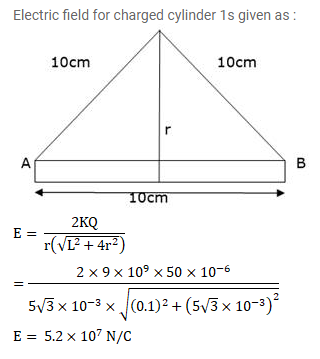# A 10 cm long rod carriers a charges of +50 μc distributed uniformly along its length.`
Question:

A $10 \mathrm{~cm}$ long rod carriers a charges of $+50 \mu \mathrm{c}$ distributed uniformly along its length. Find the magnitude of the electric field at a point $10 \mathrm{~cm}$ from both the ends of the rods.

Solution: